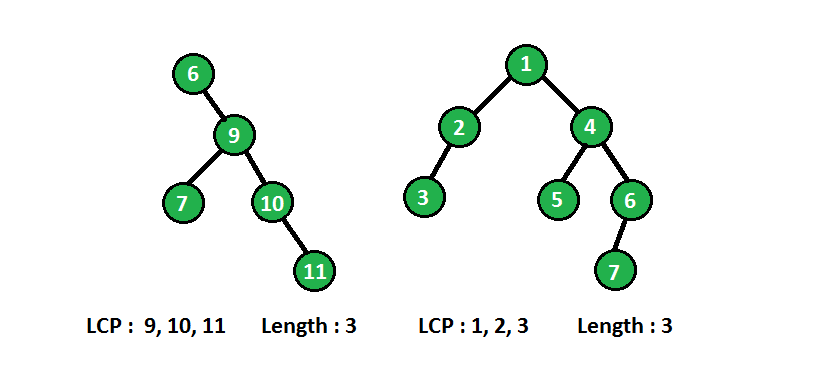Skip to content
Related Articles
Longest consecutive sequence in Binary tree
• Difficulty Level : Medium
• Last Updated : 24 Jun, 2019

Given a Binary Tree find the length of the longest path which comprises of nodes with consecutive values in increasing order. Every node is considered as a path of length 1.

Examples:

```In below diagram binary tree with longest consecutive path(LCP) are shown :```

## Recommended: Please solve it on “PRACTICE” first, before moving on to the solution.

We can solve above problem recursively. At each node we need information of its parent node, if current node has value one more than its parent node then it makes a consecutive path, at each node we will compare node’s value with its parent value and update the longest consecutive path accordingly.
For getting the value of parent node, we will pass the (node_value + 1) as an argument to the recursive method and compare the node value with this argument value, if satisfies, update the current length of consecutive path otherwise reinitialize current path length by 1.
Please see below code for better understanding :

## C++

 `// C/C++ program to find longest consecutive``// sequence in binary tree``#include ``using` `namespace` `std;`` ` `/* A binary tree node has data, pointer to left``   ``child and a pointer to right child */``struct` `Node``{``    ``int` `data;``    ``Node *left, *right;``};`` ` `// A utility function to create a node``Node* newNode(``int` `data)``{``    ``Node* temp = ``new` `Node;``    ``temp->data = data;``    ``temp->left = temp->right = NULL;``    ``return` `temp;``}`` ` `// Utility method to return length of longest``// consecutive sequence of tree``void` `longestConsecutiveUtil(Node* root, ``int` `curLength,``                              ``int` `expected, ``int``& res)``{``    ``if` `(root == NULL)``        ``return``;`` ` `    ``// if root data has one more than its parent``    ``// then increase current length``    ``if` `(root->data == expected)``        ``curLength++;``    ``else``        ``curLength = 1;`` ` `    ``//  update the maximum by current length``    ``res = max(res, curLength);`` ` `    ``// recursively call left and right subtree with``    ``// expected value 1 more than root data``    ``longestConsecutiveUtil(root->left, curLength,``                           ``root->data + 1, res);``    ``longestConsecutiveUtil(root->right, curLength,``                           ``root->data + 1, res);``}`` ` `// method returns length of longest consecutive``// sequence rooted at node root``int` `longestConsecutive(Node* root)``{``    ``if` `(root == NULL)``        ``return` `0;`` ` `    ``int` `res = 0;`` ` `    ``//  call utility method with current length 0``    ``longestConsecutiveUtil(root, 0, root->data, res);`` ` `    ``return` `res;``}`` ` `//  Driver code to test above methods``int` `main()``{``    ``Node* root = newNode(6);``    ``root->right = newNode(9);``    ``root->right->left = newNode(7);``    ``root->right->right = newNode(10);``    ``root->right->right->right = newNode(11);`` ` `    ``printf``(``"%d\n"``, longestConsecutive(root));``    ``return` `0;``}`

## Java

 `// Java program to find longest consecutive ``// sequence in binary tree`` ` `class` `Node``{``    ``int` `data;``    ``Node left, right;`` ` `    ``Node(``int` `item)``    ``{``        ``data = item;``        ``left = right = ``null``;``    ``}``}`` ` `class` `Result ``{``    ``int` `res = ``0``;``}`` ` `class` `BinaryTree``{``    ``Node root;`` ` `    ``// method returns length of longest consecutive ``    ``// sequence rooted at node root ``    ``int` `longestConsecutive(Node root)``    ``{``        ``if` `(root == ``null``)``            ``return` `0``;`` ` `        ``Result res = ``new` `Result();``         ` `        ``// call utility method with current length 0 ``        ``longestConsecutiveUtil(root, ``0``, root.data, res);``         ` `        ``return` `res.res;``    ``}`` ` `    ``// Utility method to return length of longest ``    ``// consecutive sequence of tree ``    ``private` `void` `longestConsecutiveUtil(Node root, ``int` `curlength, ``                                        ``int` `expected, Result res)``    ``{``        ``if` `(root == ``null``)``            ``return``;`` ` `        ``// if root data has one more than its parent ``        ``// then increase current length ``        ``if` `(root.data == expected)``            ``curlength++;``        ``else``            ``curlength = ``1``;`` ` `        ``// update the maximum by current length ``        ``res.res = Math.max(res.res, curlength);`` ` `        ``// recursively call left and right subtree with ``        ``// expected value 1 more than root data ``        ``longestConsecutiveUtil(root.left, curlength, root.data + ``1``, res);``        ``longestConsecutiveUtil(root.right, curlength, root.data + ``1``, res);``    ``}`` ` `    ``// Driver code``    ``public` `static` `void` `main(String args[]) ``    ``{``        ``BinaryTree tree = ``new` `BinaryTree();`` ` `        ``tree.root = ``new` `Node(``6``);``        ``tree.root.right = ``new` `Node(``9``);``        ``tree.root.right.left = ``new` `Node(``7``);``        ``tree.root.right.right = ``new` `Node(``10``);``        ``tree.root.right.right.right = ``new` `Node(``11``);`` ` `        ``System.out.println(tree.longestConsecutive(tree.root));``    ``}``}`` ` `// This code is contributed by shubham96301`

## Python3

 `# Python3 program to find longest consecutive ``# sequence in binary tree `` ` `# A utility class to create a node ``class` `newNode:``    ``def` `__init__(``self``, data):``        ``self``.data ``=` `data ``        ``self``.left ``=` `self``.right ``=` `None`` ` `# Utility method to return length of ``# longest consecutive sequence of tree ``def` `longestConsecutiveUtil(root, curLength, ``                           ``expected, res):``    ``if` `(root ``=``=` `None``):``        ``return`` ` `    ``# if root data has one more than its ``    ``# parent then increase current length ``    ``if` `(root.data ``=``=` `expected): ``        ``curLength ``+``=` `1``    ``else``:``        ``curLength ``=` `1`` ` `    ``# update the maximum by current length ``    ``res[``0``] ``=` `max``(res[``0``], curLength)`` ` `    ``# recursively call left and right subtree ``    ``# with expected value 1 more than root data ``    ``longestConsecutiveUtil(root.left, curLength, ``                           ``root.data ``+` `1``, res) ``    ``longestConsecutiveUtil(root.right, curLength,``                           ``root.data ``+` `1``, res)`` ` `# method returns length of longest consecutive ``# sequence rooted at node root ``def` `longestConsecutive(root):``    ``if` `(root ``=``=` `None``): ``        ``return` `0`` ` `    ``res ``=` `[``0``]`` ` `    ``# call utility method with current length 0 ``    ``longestConsecutiveUtil(root, ``0``, root.data, res) `` ` `    ``return` `res[``0``]`` ` `# Driver Code``if` `__name__ ``=``=` `'__main__'``:`` ` `    ``root ``=` `newNode(``6``) ``    ``root.right ``=` `newNode(``9``) ``    ``root.right.left ``=` `newNode(``7``) ``    ``root.right.right ``=` `newNode(``10``) ``    ``root.right.right.right ``=` `newNode(``11``) `` ` `    ``print``(longestConsecutive(root))`` ` `# This code is contributed by PranchalK`

## C#

 `// C# program to find longest consecutive ``// sequence in binary tree ``using` `System; ``class` `Node ``{ ``    ``public` `int` `data; ``    ``public` `Node left, right; `` ` `    ``public` `Node(``int` `item) ``    ``{ ``        ``data = item; ``        ``left = right = ``null``; ``    ``} ``} `` ` `class` `Result ``{ ``    ``public` `int` `res = 0; ``} `` ` `class` `GFG ``{ ``    ``Node root; `` ` `    ``// method returns length of longest consecutive ``    ``// sequence rooted at node root ``    ``int` `longestConsecutive(Node root) ``    ``{ ``        ``if` `(root == ``null``) ``            ``return` `0; `` ` `        ``Result res = ``new` `Result(); ``         ` `        ``// call utility method with current length 0 ``        ``longestConsecutiveUtil(root, 0, root.data, res); ``         ` `        ``return` `res.res; ``    ``} `` ` `    ``// Utility method to return length of longest ``    ``// consecutive sequence of tree ``    ``private` `void` `longestConsecutiveUtil(Node root, ``int` `curlength, ``                                        ``int` `expected, Result res) ``    ``{ ``        ``if` `(root == ``null``) ``            ``return``; `` ` `        ``// if root data has one more than its parent ``        ``// then increase current length ``        ``if` `(root.data == expected) ``            ``curlength++; ``        ``else``            ``curlength = 1; `` ` `        ``// update the maximum by current length ``        ``res.res = Math.Max(res.res, curlength); `` ` `        ``// recursively call left and right subtree with ``        ``// expected value 1 more than root data ``        ``longestConsecutiveUtil(root.left, curlength, ``                               ``root.data + 1, res); ``        ``longestConsecutiveUtil(root.right, curlength, ``                               ``root.data + 1, res); ``    ``} `` ` `    ``// Driver code ``    ``public` `static` `void` `Main(String []args) ``    ``{ ``        ``GFG tree = ``new` `GFG(); `` ` `        ``tree.root = ``new` `Node(6); ``        ``tree.root.right = ``new` `Node(9); ``        ``tree.root.right.left = ``new` `Node(7); ``        ``tree.root.right.right = ``new` `Node(10); ``        ``tree.root.right.right.right = ``new` `Node(11); `` ` `        ``Console.WriteLine(tree.longestConsecutive(tree.root)); ``    ``} ``} `` ` `// This code is contributed by 29AjayKumar`

Output:
```3
```

Also discussed on below link:
Maximum Consecutive Increasing Path Length in Binary Tree

This article is contributed by Utkarsh Trivedi. If you like GeeksforGeeks and would like to contribute, you can also write an article using contribute.geeksforgeeks.org or mail your article to contribute@geeksforgeeks.org. See your article appearing on the GeeksforGeeks main page and help other Geeks.

Please write comments if you find anything incorrect, or you want to share more information about the topic discussed above.

Attention reader! Don’t stop learning now. Get hold of all the important DSA concepts with the DSA Self Paced Course at a student-friendly price and become industry ready.  To complete your preparation from learning a language to DS Algo and many more,  please refer Complete Interview Preparation Course.

In case you wish to attend live classes with industry experts, please refer Geeks Classes Live

My Personal Notes arrow_drop_up